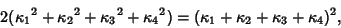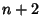## Descartes Circle Theorem

A special case of Apollonius' Problem requiring the determination of a Circle touching three mutually tangent Circles (also called the Kissing Circles Problem). There are two solutions: a small circle surrounded by the three original Circles, and a large circle surrounding the original three. Frederick Soddy gave the Formula for finding the Radius of the so-called inner and outer Soddy Circles given the Radii of the other three. The relationship iswhereare the Curvatures of the Circles. Here, the Negative solution corresponds to the outer Soddy Circle and the Positive solution to the inner Soddy Circle. This formula was known to Descartesand Viète (Boyer and Merzbach 1991, p. 159), but Soddy extended it to Spheres. In-D space,mutually touching-Spheres can always be found, and the relationship of their Curvatures isReferences

Boyer, C. B. and Merzbach, U. C. A History of Mathematics, 2nd ed. New York: Wiley, 1991.

Coxeter, H. S. M. Introduction to Geometry, 2nd ed. New York: Wiley, pp. 13-16, 1969.

Wilker, J. B. Four Proofs of a Generalization of the Descartes Circle Theorem.'' Amer. Math. Monthly 76, 278-282, 1969.